Latest Banking jobs   »

# Quantitative Aptitude Quiz For RBI Grade B Phase 1 2023 -9th March

Direction (1 – 5): In each question two equations numbered (I) and (II) are given. You should solve both equations and mark the appropriate answer.
(a) If x = y or no relation can be established
(b) If x > y
(c) If x < y
(d) If x ≥ y
(e) If x ≤ y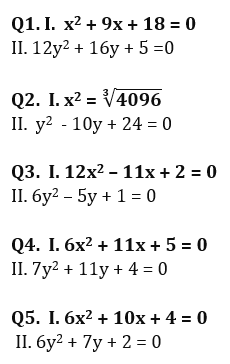Directions (6-15): In each of these questions, two equations (I) and (II) are given. Solve the equations and mark the correct option:
(a) if x>y
(b) if x≥y
(c) if x<y
(d) if x ≤y
(e) if x = y or no relation can be established between x and y.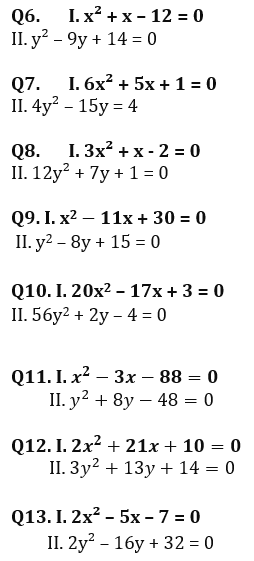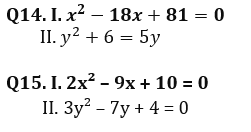Solutions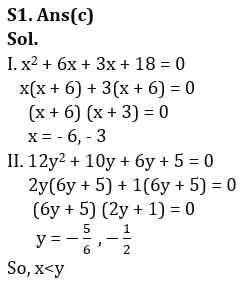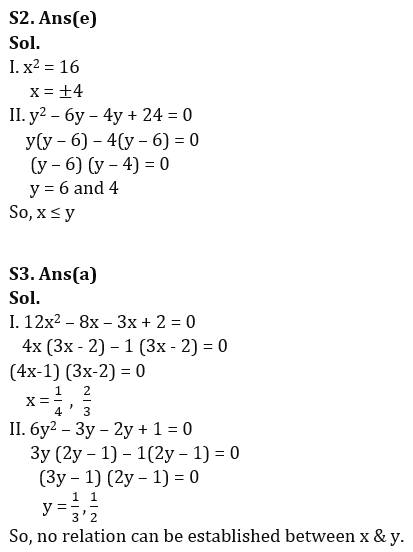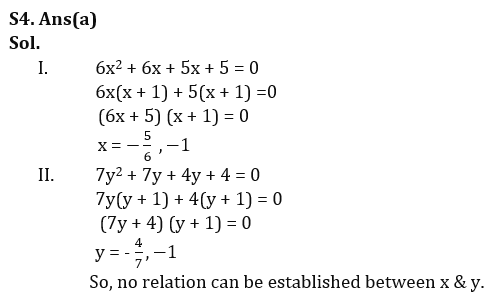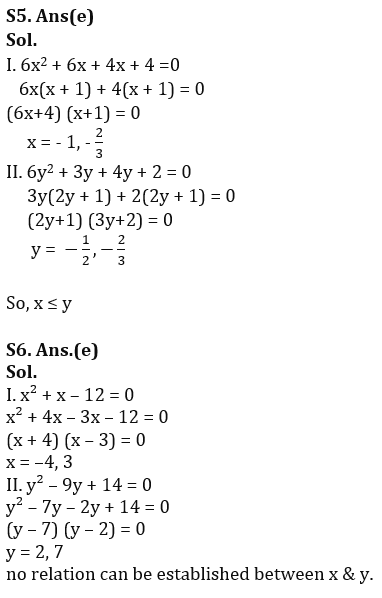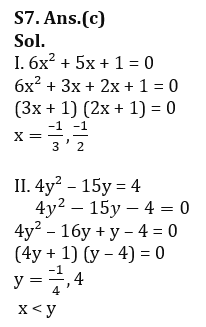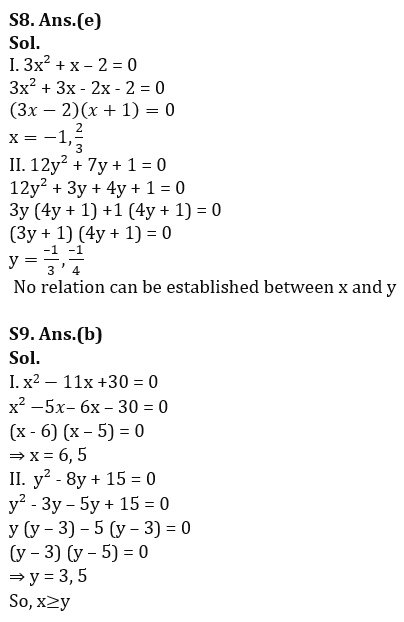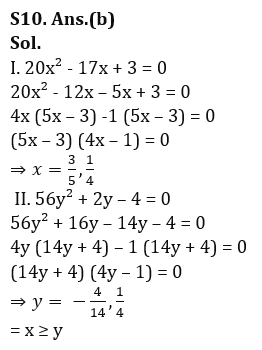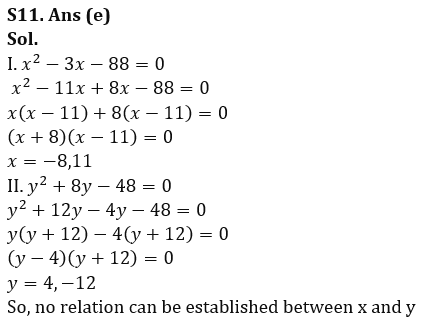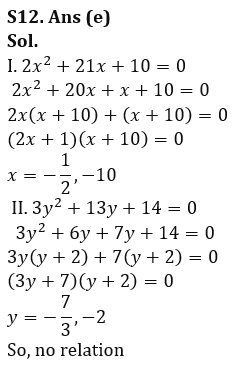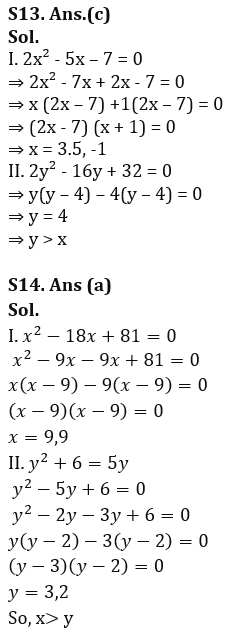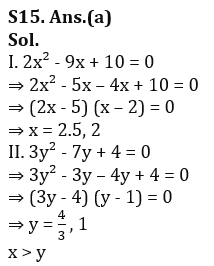.                                                                                                                                                                                                              .

## FAQs

### How many sections are there in the RBI Grade B Phase 1 Exam?

There are 4 sections in the RBI Grade B Phase 1 Exam i.e. English Language, General Awareness, Quantitative Aptitude & Reasoning.

#### Congratulations!Union Budget 2023-24: Free PDF